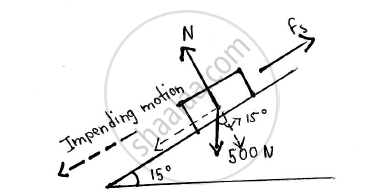Advertisement Remove all ads

# A 500 N Crate Kept on the Top of a 15° Sloping Surface is Pushed Down the Plane with an Initial Velocity of 20m/S. If μS = 0.5 and μK = 0.4, Determine the Distance Travelled by the Block and T - Engineering Mechanics

A 500 N Crate kept on the top of a 15° sloping surface is pushed down the plane with an initial velocity of 20m/s. If μs = 0.5 and μk = 0.4, determine the distance travelled by the block and the time it will take as it comes to rest.Given: Weight of crate = 500 N

Initial velocity(u) = 20 m/s

μs = 0.5

μk = 0.4

θ = 15°

Final velocity (v) = 0 m/s
To find: Distance travelled by the block Time it will take before coming to rest

Advertisement Remove all ads

#### Solution

Mass (M) W/g

500/9.81

=50.9684  kg

Normal reaction (N) on the crate = 500 cos 15

Kinetic friction (Fk) = μk x N

= 0.4xx500 cos 15

= 193.1852 N

Let T be the force down the incline Taking forces towards right of the crate as positive and forces towards left as negative

T+Fk=500 sin 15

∴ T=500 sin 15-193.1852

∴ T=-63.7756 N

By Newton’s second law of motion

a=F/m

∴ a= -63.7756/50.96684=-1.2513 m/s^2

Using kinematical equation:

v^2=u^+2as

∴ 0=202-2xx1.2513 xx s

∴ s=159.8366  m

Using kinematical equation:

V=u+at

∴ 0=20-1.2513t

∴ t=15.9837 s

 ∴ Distance travelled by the block before stopping = 159.8366 m  ∴ Time taken by the block before stopping = 15.9847 s
Concept: Velocity and Acceleration in Terms of Rectangular Co-ordinate System
Is there an error in this question or solution?
Advertisement Remove all ads

#### APPEARS IN

Advertisement Remove all ads
Advertisement Remove all ads
Share
Notifications

View all notifications

Forgot password?